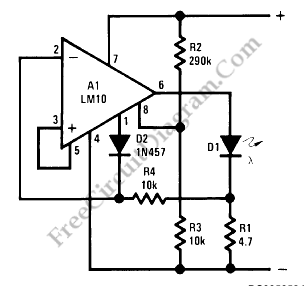# LM10 Battery Voltage Threshold IndicatorA battery threshold indicator circuit shown in the schematic diagram below has current regulation mechanism in driving the LED. A sufficient current should be satisfied at the minimum voltage but no excessive current when the voltage is at the highest level. Balance pin (5) is used as the reference  voltage for regulating the current. This pin generate 23 mV, which is internally temperature compensated.When the voltage on the reference-feedback terminal (8) drops below 200 mV, the reference output (1) rises to supply the feedback voltage to the op amp through D2, so the LED current drops to zero. The minimum threshold voltage for these circuits is basically imited by the bias voltage for the LEDs. Typically, this is 1.7V for red, 2V for green and 2.5V for yellow. These two circuits can be made to operate satisfactorily for threshold voltages as low as 2V if a red diode is used. [National Semiconductor Application Note]

This battery voltage threshold indicator circuit  overcomes the difficulties caused by voltage change across the diode biasing resistor. [Circuit’s schematic diagram source: National Semiconductor Application Note]